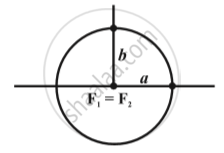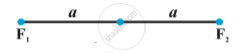CBSE (Arts) Class 11CBSE
Share

# Ellipse - Special Cases of an Ellipse

#### notes

In the equation c2 = a2 – b2 obtained above, if we keep a fixed and vary c from 0 to a, the resulting ellipses will vary in shape.
Case (i):When c = 0, both foci merge together with the centre of the ellipse and a^2 = b^2, i.e., a = b, and so the ellipse becomes circle in the above Fig.
Case (ii):When c = a, then b = 0. The ellipse reduces to the line segment F_1F_2 joining the two foci in above fig.

S